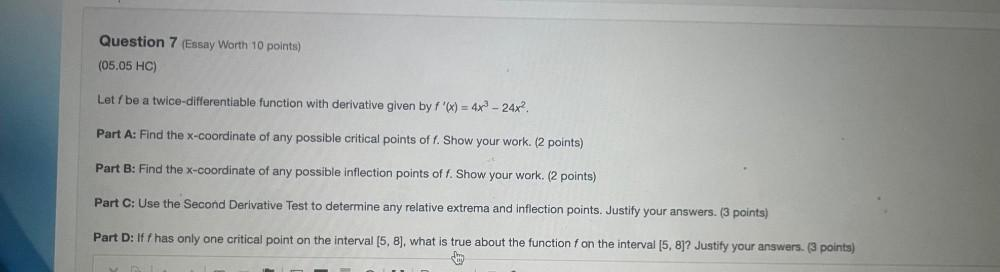Question:

# Let f be a twice-differentiable function with derivative given by f'(x) = 4x³ - 24x². (A) Find the x-coordinate of any possible critical points of f. Show your work. (B) Find the x-coordinate of any pLet f be a twice-differentiable function with derivative given by f'(x) = 4x³ - 24x². (A) Find the x-coordinate of any possible critical points of f. Show your work. (B) Find the x-coordinate of any possible inflection points of f. Show your work. (C) Use the Second Derivative Test to determine any relative extrema and inflection points. Justify your answers. (D) If f has only one critical point on the interval [5, 8], what is true about the function f on the interval [5, 8]? Justify your answers.